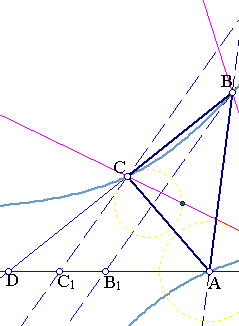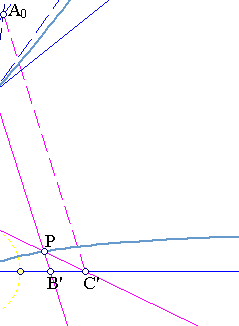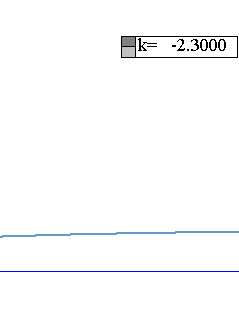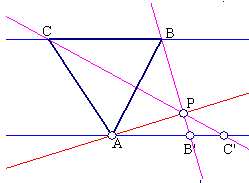##1. Chasles-Steiner example

This is an example of a Chasles-Steiner generation of a conic (see Chasles_Steiner.html ).
Consider three fixed points {A,B,C} and a fixed line AD trhough A. On line AD choose points {B',C'} such that B'A/B'C' = k is a fixed constant. The intersection points P of the variable lines {BB',CC'} generate a hyperbola.That this is a conic follows immediately by taking the origin of coordinates on AD to be at A and setting AB'=x, AC'=y, this means that -x/(y-x)=k => y=((k-1)/k)x, which is a very simple homographic relation as required by the Chasles-Steiner method.
To see that this is a hyperbola suffices to notice that it intersects the line at infinity at two distinct points. The first is determined by the direction of the line AD, since B' going to infinity implies that C' does the same and lines {BB',CC'} become parallel to AD.
The other point at infinity lying on the conic is found as follows. Note first that since B'A/B'C' is constant the parallel to BB' from C' intersects AB at a fixed point A0. Line A0C intersects AD at a point C1 and the parallel to A0C from B defines on AD a point B1 and obviously B1A/B1C1=k. Thus the other point at infinity on the conic is the point determined by the parallels {A0C1,BB1}.

##2. A special case

A special case occurs when BC is parallel to line AD. Then line AP intersects BC at a point E such that BE/BC=k, hence E is fixed and P moves on line AE (see figure below).##3. The inverse : a hyperbola property

Let {A,B,C} be three points on a hyperbola and consider the parallel to an asymptote through A line AD. Then for any point P on the hyperbola lines {PB,PC} intersect line AD at points {B',C'} such that B'A/B'C' is constant.

The proof of the property can be based on section-1. After it, forgetting for the moment the given hyperbola c, we determine another hyperbola c' describing the locus of a point P as in section-1. For this take an arbitrary point P0 on c and determine the ratio k=B'A/B'C. Construct then c' by the data {A,B,C,D,k} as above. The two hyperbolas {c,c'} coincide then on the four points {A,B,C, P0} and the point at infinity determined by line AD. Hence they are identical.

##4. Some computations in barycentrics

Let as determine the equation of the hyperbola in barycentric coordinates in terms of the given points {A,B,C}, line AD and the ratio k (see BarycentricCoordinates.html ).

In these coordinates point D (fixed) on BC has the expression D=B+pC. The variable points B'=D+mA=mA+B+pC, C'=D+nA=nA+B+pC are related by having a constant ratio B'A/B'C'=k.
By the general theory the locus of points P is a conic passing through {A,B,C} hence having the form :
Myz + Nzx + Pxy =0 (*).
In addition the curve passes through two points at infinity determined by the given conditions. The first is the point at infinity determined by line AD. This point is obtained as B' tends to this point at infinity along AD.
The second point is the point at infinity of line A0C, where A0 is the point on AB, such that BA/BA0=k (figure and section-1).

 Line AD : has coefficients given by the vector product (1,0,0)x(0,1,p)=(0,-p,1), with point at infinity (0,-p,1)x(1,1,1) =
(-1-p, 1, p).
 The second point at infinity needs point A0 which must be A0 = A+(k-1)B.
Then line CA0 has coefficients (0,0,1)x(1,(k-1),0)=(1-k,1,0) and point at infinity (k,1,0)x(1,1,1)=(1,k-1,-k).
Introducing these into (*) we obtain the equations for {M,N,P}:
(-p)M +p(1+p)N + (1+p)P = 0,
k(k-1)M +kN + (1-k)P = 0.
Obviously (M,N,P) is a multiple of the vector product (-p,p(1+p), (1+p)) x (k(k-1), k, 1-k), which leads easily to the conic equation:
(1+p)yz + (1-k)zx + (pk)xy = 0.

Remark-1 Constants {k,p} can be easily determined from {M,N,P} and lead to quadratic equations corresponding to the two possible choices of one or the other asymptote.
Remark-2 Inversely {k,p} determine the coefficients {M,N,P} of the conic and it is easily seen that these satisfy the equation P+(M-1)(N-1)=0.

### See Also

BarycentricCoordinates.html
Chasles_Steiner.html

 Produced with EucliDraw© http://users.math.uoc.gr/~pamfilos/